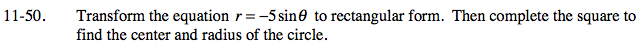Home > PC > Chapter 11 > Lesson 11.1.3 > Problem11-50

11-50.

Transform the equation r = −5 sinθ to rectangular form. Then complete the square to find the center and radius of the circle. Homework Help ✎Begin by multiplying both sides of the equation by r.